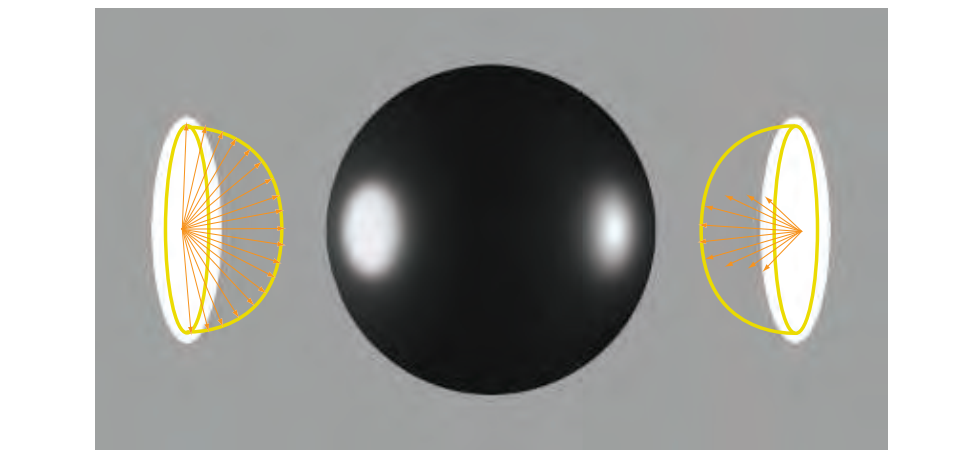"Light makes right. " — Andrew Glassner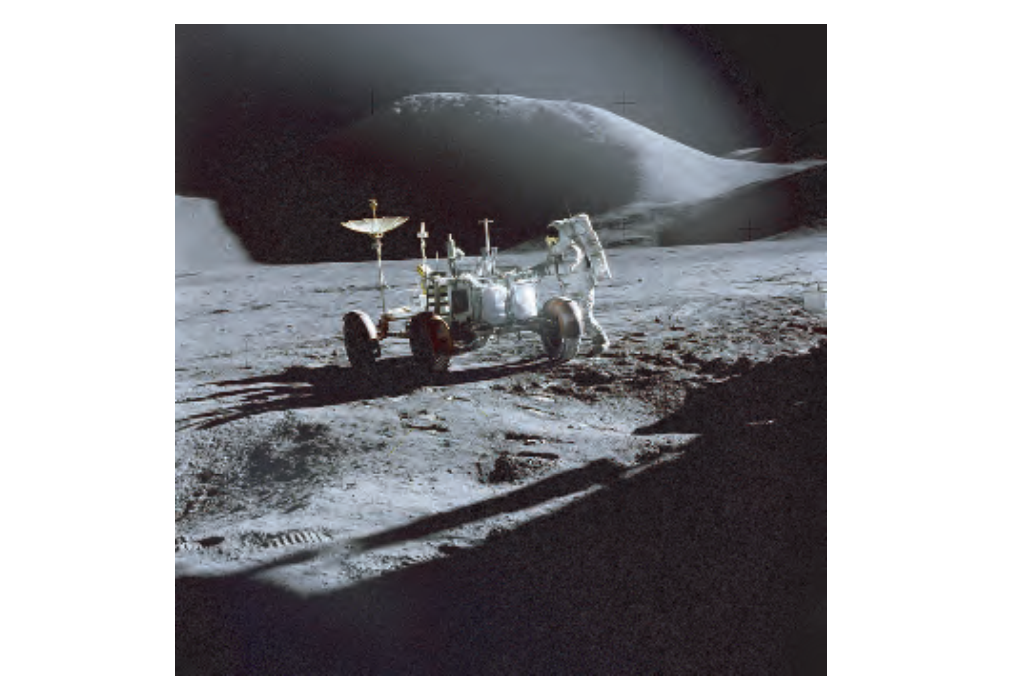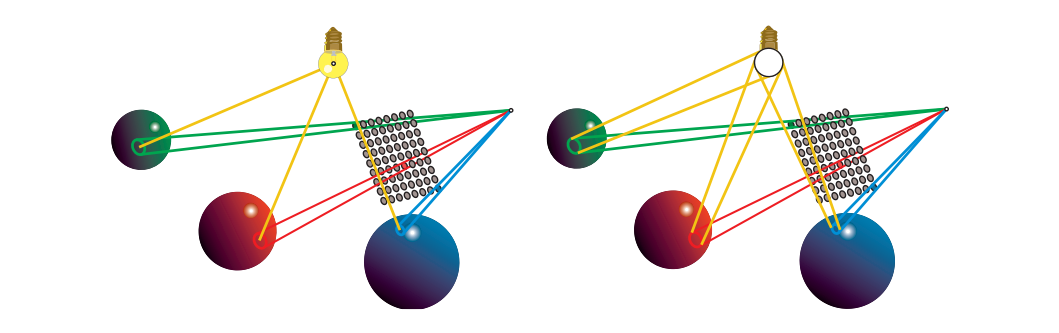### 10.1 Area Light Sources（区域光源）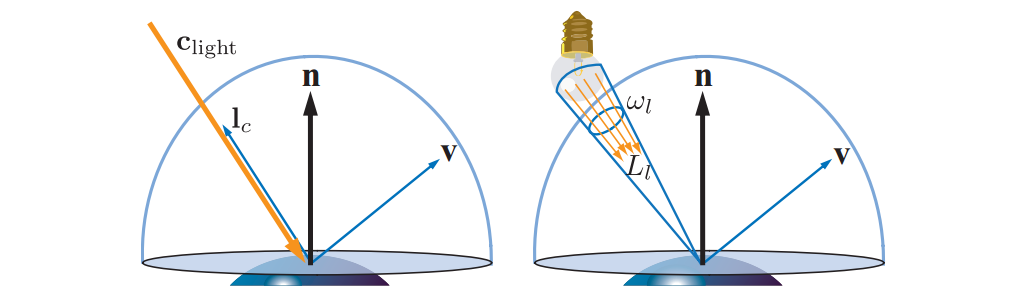$$L_o(\boldsymbol{v})=\int_{\boldsymbol{l}\in\omega_l}f(\boldsymbol{l,v})L_l(\boldsymbol{n\cdot l})^+d\boldsymbol{l}\approx\pi f(\boldsymbol{l}_c,\boldsymbol{v})\boldsymbol{c}_{light}(\boldsymbol{n\cdot l_c})^+.\tag{10.1}$$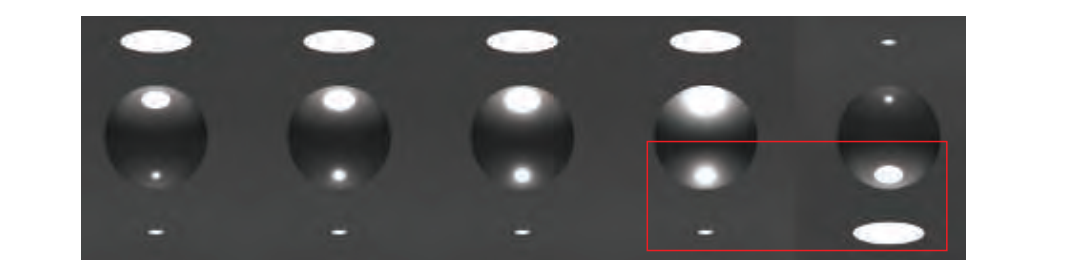$$L_o(\boldsymbol{v})=\frac{\rho_{ss}}{\pi}E,\tag{10.2}$$

$$E=\int_{\boldsymbol{l}\in\omega_l}L_l(\boldsymbol{n\cdot l})^+d\boldsymbol{l}\approx\pi\boldsymbol{c}_{light}(\boldsymbol{n}\cdot\boldsymbol{l}_c)^+.\tag{10.3}$$

$$\boldsymbol{e}(\boldsymbol{p})=\int_{\boldsymbol{l}\in\Theta}L_i(\boldsymbol{p,l})\boldsymbol{l}d\boldsymbol{l},\tag{10.4}$$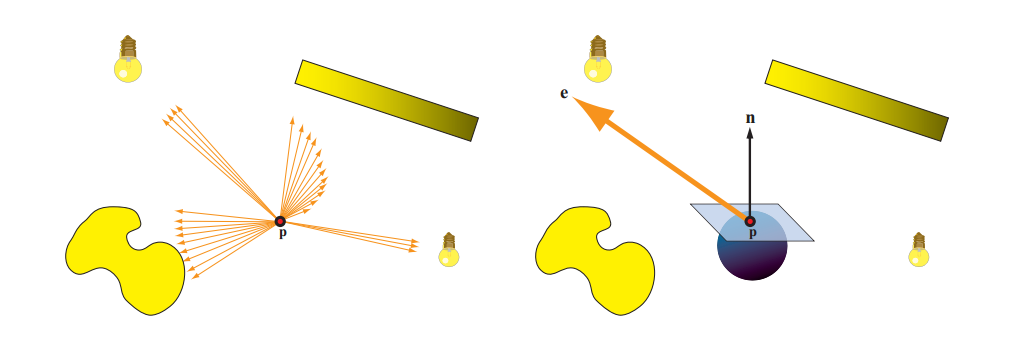$$E(\boldsymbol{p,n})-E(\boldsymbol{p,-n})=\boldsymbol{n\cdot e(p)},\tag{10.5}$$

$$E(\boldsymbol{p,n})=\boldsymbol{n\cdot e(p)},\tag{10.6}$$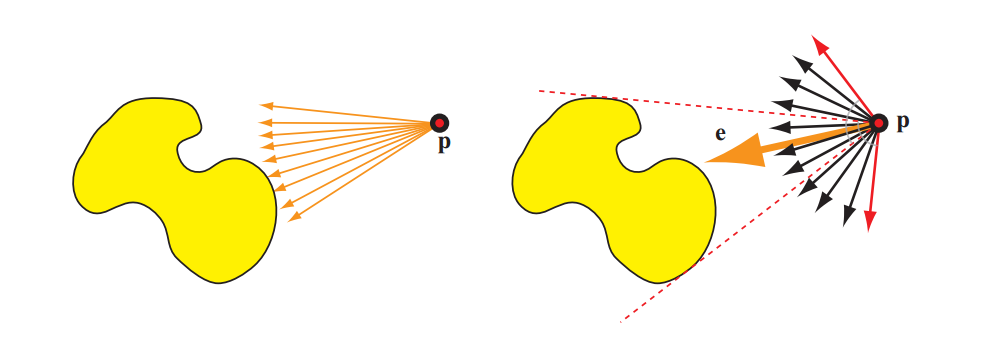\begin{align} \boldsymbol{l}_c&=\frac{\boldsymbol{e(p)}}{\Vert\boldsymbol{e(p)}\Vert},\\ \boldsymbol{c}_{light}&=\boldsymbol{c}'\frac{\Vert\boldsymbol{e(p)}\Vert}{\pi}. \end{align} \tag{10.7}

\begin{align} \boldsymbol{l}_c&=\frac{\boldsymbol{p}_l-\boldsymbol{p}}{\Vert\boldsymbol{p}_l-\boldsymbol{p}\Vert},\\ \boldsymbol{c}_{light}&=\frac{r_l^2}{\Vert\boldsymbol{p}_l-\boldsymbol{p}\Vert^2}L_l. \end{align} \tag{10.8}

$$E=\pi\boldsymbol{c}_{light}(\frac{(\boldsymbol{n\cdot l})+k_{wrap}}{1+k_{wrap}})^+,\tag{10.9}$$

$$E=\pi\boldsymbol{c}_{light}(\frac{(\boldsymbol{n\cdot l})+1}{2})^2,\tag{10.10}$$

#### 10.1.1 Glossy Materials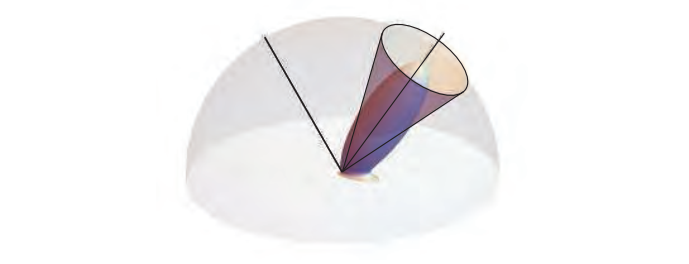Karis展示了Mittring原理在GGX / Trowbridge-Reitz BRDF（第9.8.1节）和球面光源中的应用，导致GGX粗糙度参数 $α_g$ 的简单修改：

$$\alpha_g'=(\alpha_g+\frac{r_l}{2\Vert\boldsymbol{p}_l-\boldsymbol{p}\Vert})^\mp$$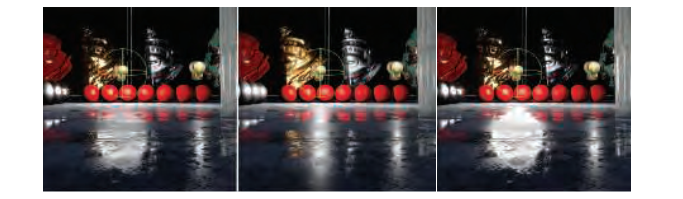$$\int_Df(x)dx=f(c)\int_D1.\tag{10.11}$$

#### 10.1.2 General Light Shapes（常见的光源形状）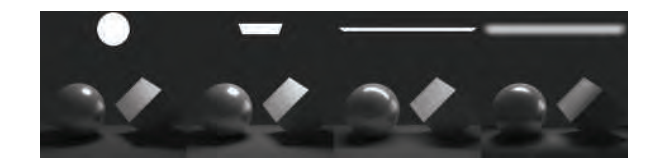$$\int^{\boldsymbol{p}_1}_{\boldsymbol{p}_0}(\boldsymbol{n}\cdot\frac{x}{\Vert x\Vert})\frac{1}{\Vert x\Vert^2}dx=\frac{\frac{\boldsymbol{n\cdot p_o}}{\Vert\boldsymbol{p_0}\Vert^2}+\frac{\boldsymbol{n\cdot p_1}}{\Vert\boldsymbol{p_1}\Vert^2}}{\Vert\boldsymbol{p_0}\Vert\Vert\boldsymbol{p_1}\Vert+(\boldsymbol{p_0}\cdot\boldsymbol{p_1})},\tag{10.12}$$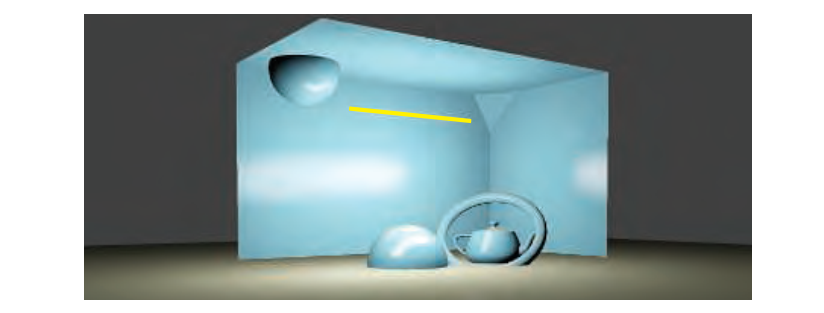Drobot推出了card light（以及disk）的第一个实用近似值。这又是一个代表性的点解决方案，但是由于将该方法扩展到平面的二维区域以及解决方案的整体方法的复杂性，这尤其值得注意。Drobot从均值定理开始，并且作为第一近似，确定用于光评估的良好候选点应该位于照明积分的全局最大值附近。

$$L_l\int_{\boldsymbol{l}\in\omega_l}(\boldsymbol{n\cdot l})^+\frac1{r^2_\boldsymbol{l}}d\boldsymbol{l},\tag{10.13}$$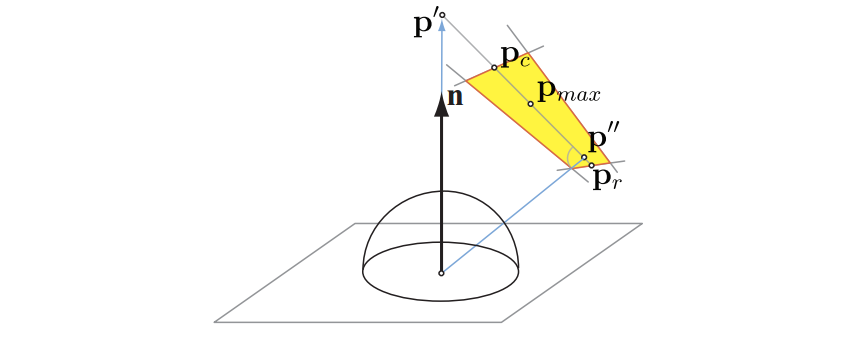Drobot的最终解决方案采用了漫反射和高光照明的进一步近似，所有这些都是通过与数字上发现的地面实况解决方案进行比较来实现的。他还为纹理卡片灯的重要案例推导出一种算法，其中发射不被认为是恒定的，而是通过纹理在光的矩形区域上调制。使用三维查找表来执行该过程，该查找表包含在不同半径的圆形足迹上的发射纹理的预集成版本。Mittring采用类似的方法进行光泽反射，将反射光线与纹理矩形广告牌相交，并根据光线交叉距离索引预先计算的纹理模糊版本。这项工作先于Drobot的发展，但它是一种更经验，更少原则的方法，试图与地面实况完整解决方案相匹配。

### 10.2 Environment Lighting（环境光照）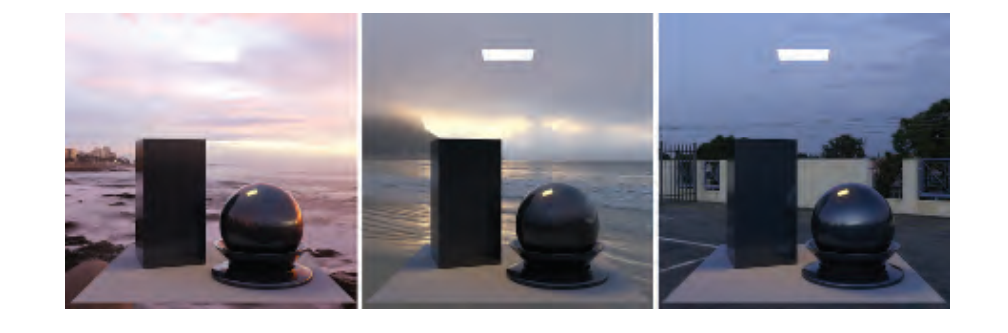$$L_o(\boldsymbol{v})=\frac{\rho_{ss}}{\pi}L_A\int_{\boldsymbol{l}\in\Omega}(\boldsymbol{n\cdot l})d\boldsymbol{l}=\rho_{ss}L_A.\tag{10.14}$$

$$L_o(\boldsymbol{v})=L_A\int_{\boldsymbol{l}\in\Omega}f(\boldsymbol{l,v})(\boldsymbol{n\cdot l})d\boldsymbol{l}.\tag{10.15}$$

### 10.3 Spherical and Hemispherical Functions（球面和半球函数）

• 高效的编码（投影）和解码（查找）。
• 使球函数表示为具有少量系数和低重建误差。
• 投影的旋转不变性是旋转函数的投影的结果，与旋转函数然后投影它相同。 这种等效意味着用例如球谐函数近似的函数在旋转时不会改变。
• 易于计算编码函数的总和和乘积。
• 易于计算球形积分和卷积。

#### 10.3.1 Simple Tabulated Forms（简单的表格形式）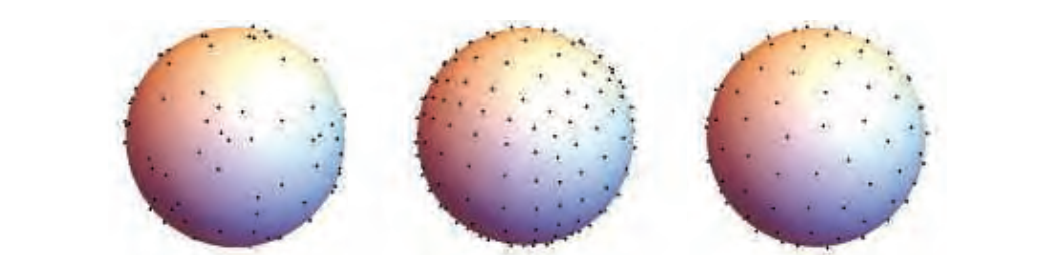$$F_{AC}(\boldsymbol{d})=\boldsymbol{d}d\cdot sel_+(\boldsymbol{c}_+,\boldsymbol{c}_-,\boldsymbol{d}),\tag{10.16}$$

#### 10.3.2 Spherical Bases（球面基础）

$$F_c(\theta,\phi)=c\cdot1.$$

$$F_{hemi}(\theta,\phi)=a+\frac{\cos(\theta)+1}2(b-a),$$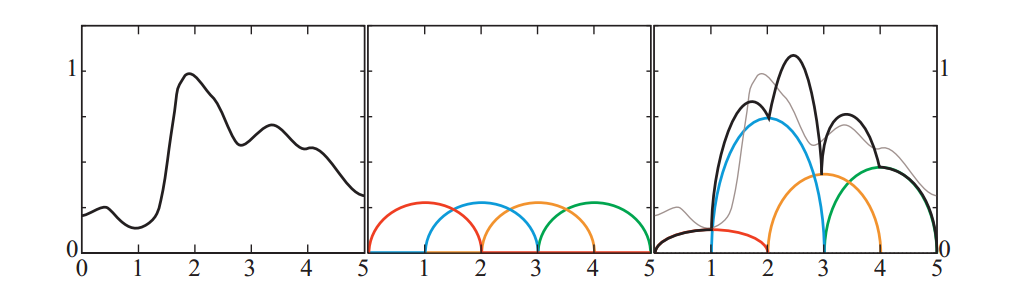##### Spherical Gaussians

SRBF波瓣的一个特别常见的选择是球面高斯（SG），在方向统计中也称为von Mises-Fisher分布。我们应该注意到von-Mises-Fisher分布通常包括归一化常数，我们在公式中避免使用。单个lobe可以定义为：

$$G(\boldsymbol{v,d},\lambda)=e^{\lambda(\boldsymbol{v\cdot d}-1)},\tag{10.17}$$

$$F_G(\boldsymbol{v})=\sum_kw_kG(\boldsymbol{v,d_k},\lambda_k).\tag{10.18}$$

$$G_1G_2=G(\boldsymbol{v},\frac{\boldsymbol{d}'}{\Vert\boldsymbol{d}'\Vert},\lambda'),$$

where:

$$\boldsymbol{d}'=\frac{\lambda_1\boldsymbol{d}_1+\lambda_2\boldsymbol{d}_2}{\lambda_1+\lambda_2},;;\lambda'=(\lambda_1+\lambda_2)\Vert\boldsymbol{d}'\Vert.$$

$$\int_\Omega G(\boldsymbol{v})d\boldsymbol{v}=2\pi\frac{1-e^{2\lambda}}{\lambda},$$

$$G(\boldsymbol{v},[\boldsymbol{d,t,b}],[\lambda,\mu])=S(\boldsymbol{v,d})e^{-\lambda(\boldsymbol{v\cdot t})^2-\mu(\boldsymbol{v\cdot b})^2},\tag{10.19}$$

Spherical Harmonics（球谐波）

$$\langle f_i(x),f_j(x) \rangle \equiv \int f_i(x)f_j(x)dx,\tag{10.20}$$

$$\langle f_i(\boldsymbol{n}),f_j(\boldsymbol{n}) \rangle \equiv \int_{\boldsymbol{n}\in\Theta} f_i(\boldsymbol{n})f_j(\boldsymbol{n})d\boldsymbol{n},\tag{10.21}$$

$$\langle f_i(),f_j() \rangle = \begin{cases} 0, ;where ;i\ne j,\\ 1, ;where ;i= j. \end{cases} \tag{10.22}$$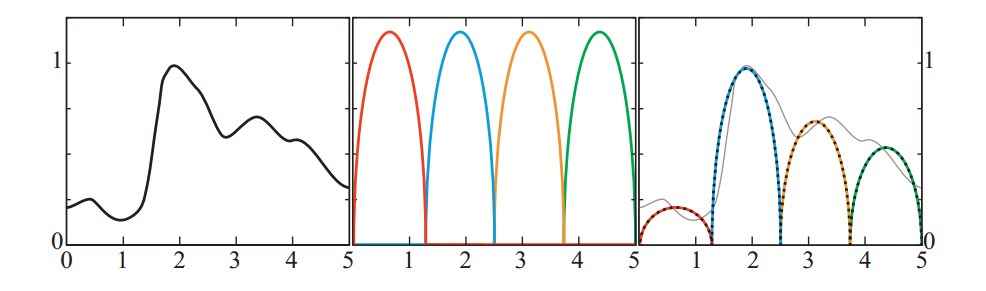$$k_j=\langle f_{target}(x),f_j(x) \rangle,\\ f_{target}(x)\approx\sum_{j=1}^nk_jf_j().\tag{10.23}$$

SH基函数排列在频带中。第一个基函数是常数，接下来的三个是在球体上缓慢变化的线性函数，接下来的五个函数表示稍微更快变化的二次函数。见图10.21。低频率（即在球体上缓慢变化）的函数，例如辐照度值，用相对较少数量的SH系数精确表示（我们将在第10.6.1节中看到）。



#### 10.3.3 Hemispherical Bases

##### Hemispherical Harmonics/H-Basis

hemispherical harmonics (HSHs) ...暂略

### 10.4 Environment Mapping

$$\boldsymbol{r}=2(\boldsymbol{n\cdot v})\boldsymbol{n}-\boldsymbol{v}.\tag{10.28}$$

$$L_o(\boldsymbol{v})=F(\boldsymbol{n,r})L_i(\boldsymbol{r}),\tag{10.29}$$

reflection mapping 算法的步骤如下：

• 生成/加载表示环境的纹理。
• 对于每个包含反射对象的像素，计算该对象表面位置的法线。
• 从v和n计算reflected view vector 。
• 使用reflected view vector 计算环境贴图中的索引，该索引表示反射视图方向上的入射辐射。
• 使用环境贴图中的纹素数据作为公式10.29中的入射辐射。

#### 10.4.1 Latitude-Longitude Mapping

1976年，Blinn和Newell开发了第一个环境映射算法。他们使用的映射是在地球上使用的熟悉的纬度/经度系统，这就是为什么这种技术通常被称为纬度 - 经度映射或纬度 - 长度映射的原因。他们的计划不像是从外面看的地球，而是像夜空中的星座图。 就像地球上的信息可以展平为墨卡托或其他投影地图一样，可以将围绕空间中的点的环境映射到纹理。 当针对特定表面位置计算反射视图矢量时，矢量被转换为球面坐标 $(ρ,\phi)$ 。这里的φ，相当于经度，从0到2π弧度变化，ρ，纬度，从0到π弧度变化。 对 $(ρ,\phi)$ 由公式10.30计算，其中 $\boldsymbol{r} =(\boldsymbol{r}_x, \boldsymbol{r}_y, \boldsymbol{r}_z)$ 是归一化的反射视图向量，其中+ z为上方向：

$$\rho=arccos(r_z);and;\phi=atan2(r_y,r_x).\tag{10.30}$$#### 10.4.2 Sphere Mapping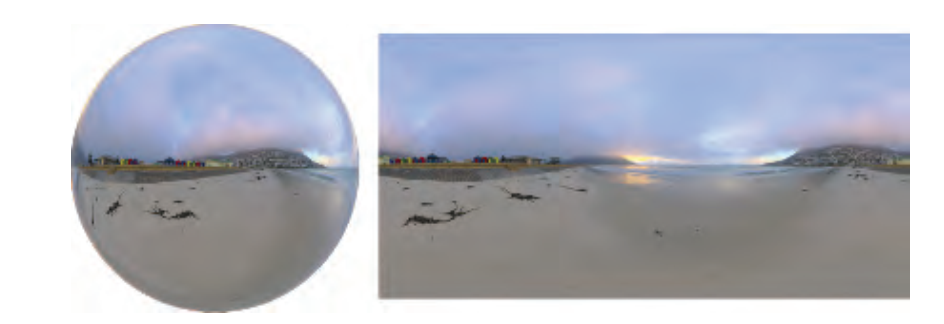$$\boldsymbol{n}=(\frac{r_x}m,\frac{r_y}m,\frac{r_z+1}m),;where;m=\sqrt{r_x^2+r_y^2+(r_z + 1)^2}.\tag{10.31}$$

$$m=\sqrt{r_x^2+r_y^2+(r_z + 1)^2},;u=\frac{r_x}{2m}+0.5,;and;v=\frac{r_y}{2m}+0.5.\tag{10.32}$$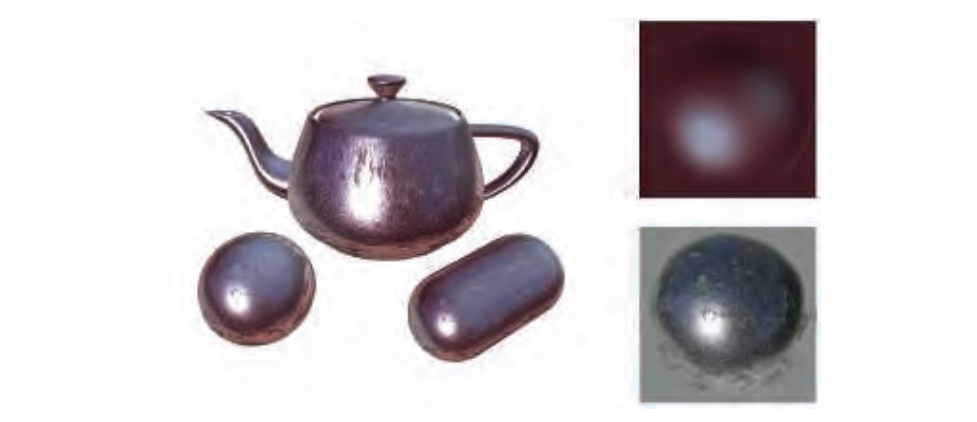#### 10.4.3 Cube Mapping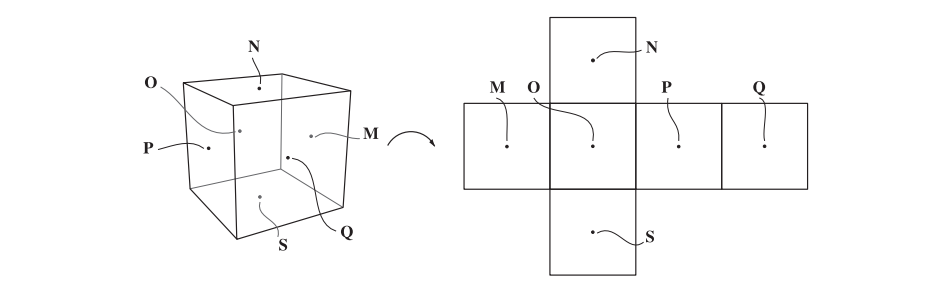1986年，Greene推出了立方体环境地图，通常称为立方体地图。 这种方法是当今最流行的方法，其投影直接在现代GPU上的硬件中实现。 通过将环境投影到立方体的侧面来创建立方体贴图，该立方体的中心位于相机的位置。 然后将立方体面上的图像用作环境贴图。 立方体贴图通常在“交叉”图中可视化，即打开立方体并将其展平到平面上。但是，在硬件立方体贴图上存储为六个方形纹理，而不是单个矩形纹理，因此不会浪费空间。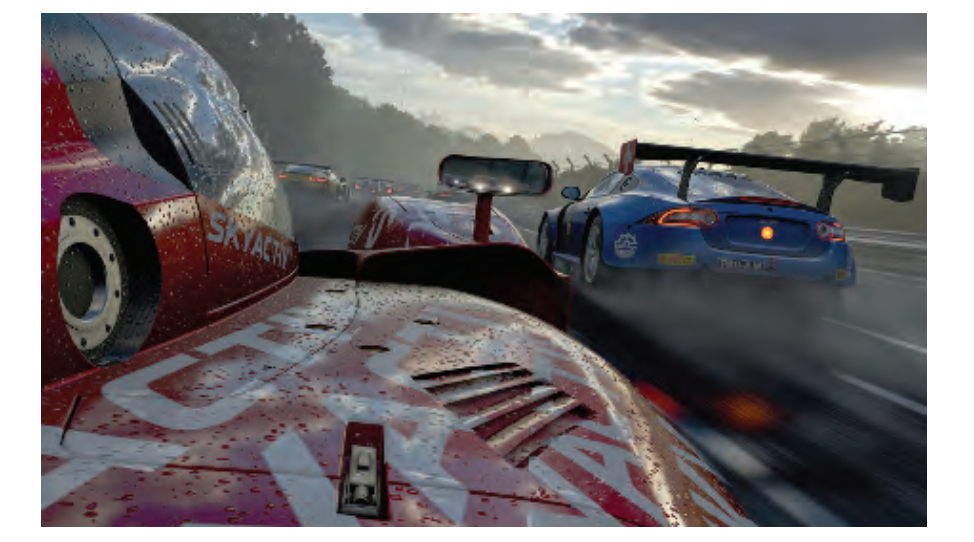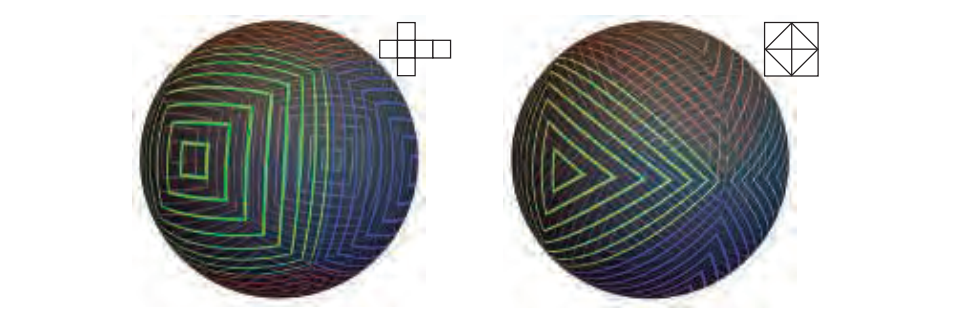#### 10.4.4 Other Projections

Heidrich和Seidel建议使用两个纹理来执行双抛物面环境映射（dual paraboloid environment mapping）。这个想法就像是球体映射的想法，但不是通过记录球体环境的反射来生成纹理，而是使用两个抛物线投影。每个抛物面创建一个类似于球体地图的圆形纹理，每个抛物面覆盖一个环境半球。

$$u=\frac{r_x}{2(1+r_z)}+0.5,;v=\frac{r_y}{2(1+r_z)}+0.5\tag{10.33}$$

$$\boldsymbol{r'}=\frac{\boldsymbol{r}}{\vert r_x\vert+\vert r_y\vert+\vert r_z\vert}.$$

$$u=r_x'\cdot0.5+0.5,v=r_y'\cdot0.5+0.5.\tag{10.34}$$

$$u=(1-\vert r'_z\vert)\cdot sign(r_x')\cdot0.5+0.5,;v=(1-\vert r_x'\vert)\cdot sign(r'_z)\cdot0.5+0.5.\tag{10.35}$$

### 10.5 Specular Image-Based Lighting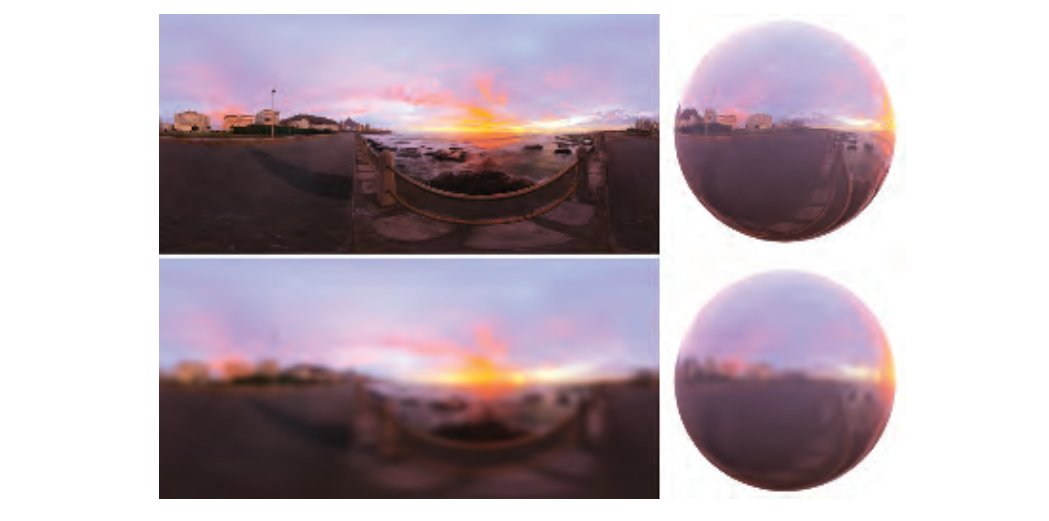#### 10.5.1 Prefiltered Environment Mapping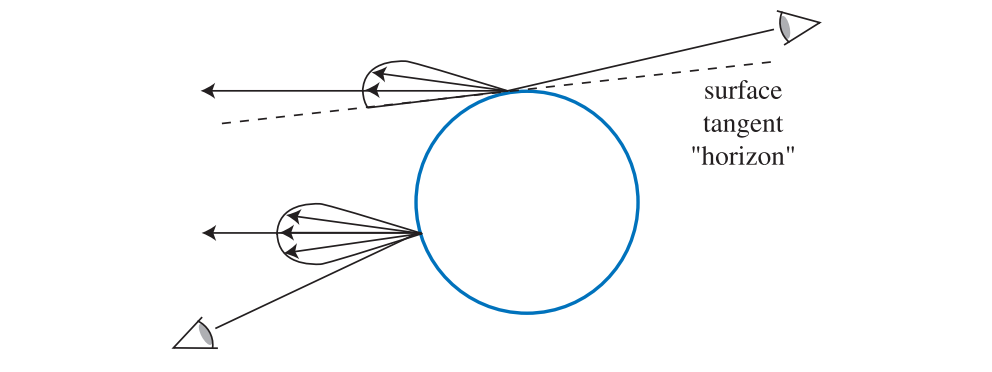Heidrich和Seidel 以这种方式使用单个反射贴图来模拟表面的模糊度。为了适应不同的粗糙度级别，通常使用环境立方体贴图的mipmap（第6.2.2节）。每个级别用于存储传入辐射的模糊版本，较高的mip级别存储较粗糙的表面，即较宽的Phong波瓣。在运行期间，我们可以通过使用反射向量来解决立方体贴图，并根据所需的Phong指数（材料粗糙度）强制选择给定的mip级别。见图10.36。##### Convolving the Environment Map（卷积环境映射）

$$\int_\Omega D(\boldsymbol{l,v})L_i(\boldsymbol{l})d\boldsymbol{l}.$$

$$\int_\Omega D(\boldsymbol{l,v})L_i(\boldsymbol{l})d\boldsymbol{l}\approx\lim_{N\to\infin}\frac1N\sum^N_{k=1}\frac{D(\boldsymbol{l_k,v})L_i(\boldsymbol{l}_k)}{p(\boldsymbol{l}_k,\boldsymbol{v})},\tag{10.36}$$

Kautz等人呈现另一种变型，用于快速生成滤波抛物面反射映射的分层技术。最近，Manson和Sloan使用有效的二次B样条滤波方案显着改进了现有技术，以生成环境地图的mip级别。这些特殊计算的B样条滤波mips然后通过组合几个样本来使用，其方式与McGuire等人和Kautz等人的技术类似，以产生快速和准确的近似。这样做可以实时生成与通过重要性采样蒙特卡罗技术计算的地面实况无法区分的结果。

#### 10.5.2 Split-Integral Approximation for Microfacet BRDFs （微面BRDF的分步积分近似）

$$\int_{\boldsymbol{l}\in\Omega}f(\boldsymbol{l,v})L_i(\boldsymbol{l})(\boldsymbol{n\cdot l})d\boldsymbol{l}\approx F(\boldsymbol{n,v})\int_\boldsymbol{l\in\Omega}D_{Phong}(\boldsymbol{r})L_i(\boldsymbol{l})(\boldsymbol{n\cdot l})d\boldsymbol{l},\tag{10.37}$$

$$f_{smf}(\boldsymbol{l,v})=\frac{F(\boldsymbol{h,l})G_2(\boldsymbol{l,v,h})D(\boldsymbol{h})}{4\vert\boldsymbol{n\cdot l}\vert\vert\boldsymbol{n\cdot v}\vert}\tag{10.38}$$

$$\int_\boldsymbol{l\in\Omega}f_{smf}(\boldsymbol{l,v})L_i(\boldsymbol{l})(\boldsymbol{n\cdot l})d\boldsymbol{l}\approx\int_{\boldsymbol{l\in\Omega}}D(\boldsymbol{r})L_i(\boldsymbol{l})(\boldsymbol{n\cdot l})d\boldsymbol{l}\int_{\boldsymbol{l\in\Omega}}f_{smf}(\boldsymbol{l,v})(\boldsymbol{n\cdot l})d\boldsymbol{l}.\tag{10.39}$$

#### 10.5.3 Asymmetric and Anisotropic Lobes（不对称和各向异性的Lobes）

Kautz和McCool通过使用存储在预滤波环境图中的径向对称叶片的更好的采样方案来改进之前的预积分。他们提出了两种方法。第一个使用单个样本，但试图找到最好的波瓣在当前视图方向上近似BRDF，而不是依赖于恒定的校正因子。第二种方法平均来自不同叶片的几个样品。第一种方法更好地模拟掠射角度的表面。它们还导出校正因子以解释使用径向对称波瓣近似与原始BRDF相比反射的总能量的差异。第二种解决方案将结果扩展到包括半矢量模型典型的拉伸高光。在这两种情况下，优化技术用于计算驱动预滤波叶片采样的参数表。Kautz和McCool的技术使用贪婪拟合算法和抛物线环境贴图。

McAllister等开发了一种技术，通过利用Lafortune BRDF的特性，能够产生各种效果，包括各向异性和逆向反射。该BRDF 本身是基于物理的渲染的近似。它由多个Phong叶片组成，围绕反射方向扰动。 Lafortune证明了这种BRDF能够通过将这些叶片拟合到He-Torrance模型以及从gonioreflectometer测量真实材料来表示复杂材料。McAllister的技术依赖于注意到，由于Lafortune叶片是广义Phong叶片，因此可以使用传统的预滤波环境图，其mips编码不同的Phong指数。格林等人提出了一种类似的方法，其使用高斯波瓣而不是Phong波瓣。此外，他们的方法可以扩展到支持环境地图的方向阴影（第11.4节）。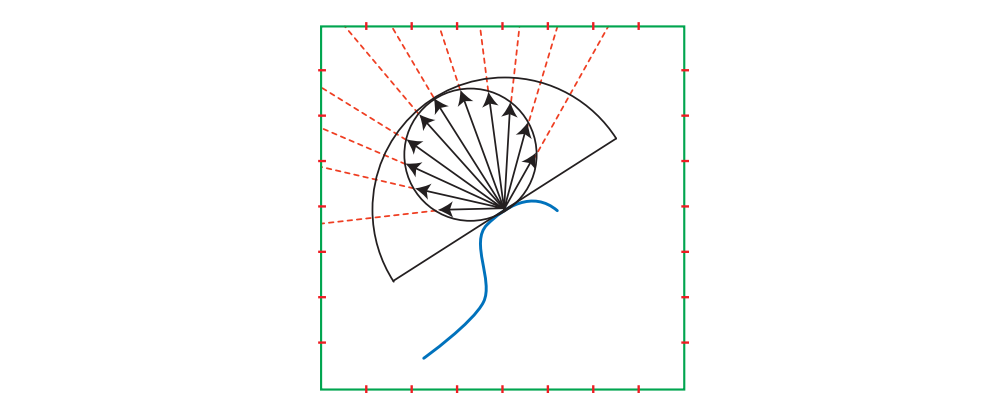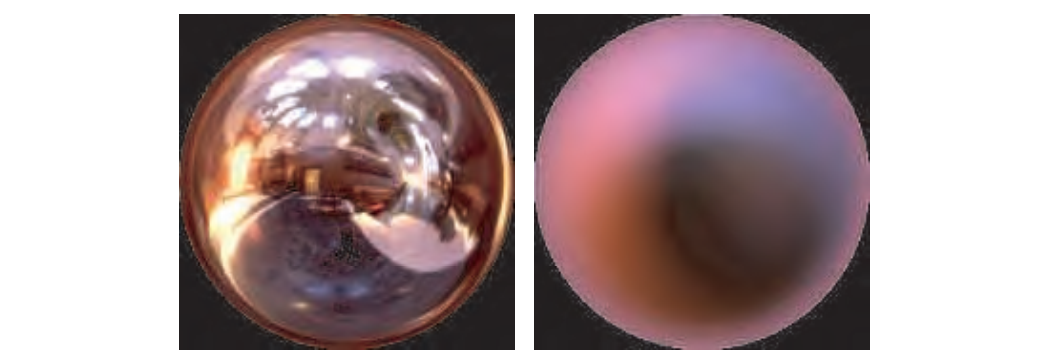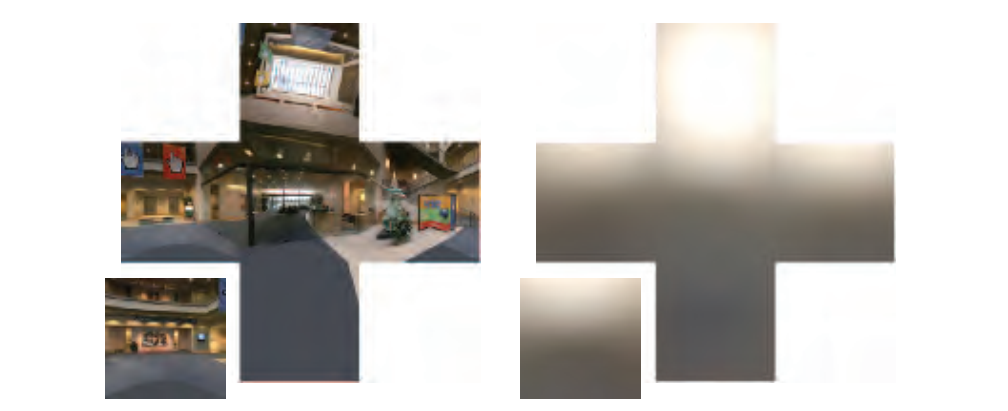#### 10.6.1 Spherical Harmonics Irradiance （球谐函数辐照度）

Ramamoorthi和Hanrahan表明，只用前九个SH系数（每个系数是RGB矢量，因此我们需要存储27个浮点数），辐照度环境图可以表示为误差在1％的精度。然后可以将任何辐照度环境图解释为球函数 $E(\boldsymbol{n})$ 并使用等式10.21和10.23投影到九个RGB系数上。这种形式比立方体或抛物线图更紧凑，在渲染过程中，可以通过计算一些简单的多项式来重建辐照度，而不是访问纹理。通常，如果辐照度环境图表示间接照明（交互式应用中的常见情况），则需要较低的精度。在这种情况下，对于恒定基函数和三个线性基函数，四个系数通常可以产生良好的结果，因为间接照明倾向于低频，即随角度缓慢变化。

Ramamoorthi和Hanrahan 还表明，通过将每个系数乘以常数，可以将入射辐射函数 $L(\boldsymbol{l})​$ 的SH系数转换成辐照度函数 $E(\boldsymbol{n})​$ 的系数。这样做可以快速地将环境贴图过滤到辐照度环境贴图中，即将它们投影到SH基础中，然后将每个系数乘以常数。例如，这是King的快速辐照度滤波实现的工作原理。该想法是从辐射度计算辐照度等效于在进入辐射函数 $L(\boldsymbol{l})​$ 和钳位余弦函数 $cos(θ_i)^+​$之间执行球形卷积。由于钳位余弦函数关于球体的z轴旋转对称，因此它在SH中呈现特殊形式：其投影在每个频带中仅具有一个非零系数。非零系数对应于图10.21（第401页）中间列中的基函数，也称为区域谐波（zonal harmonics）。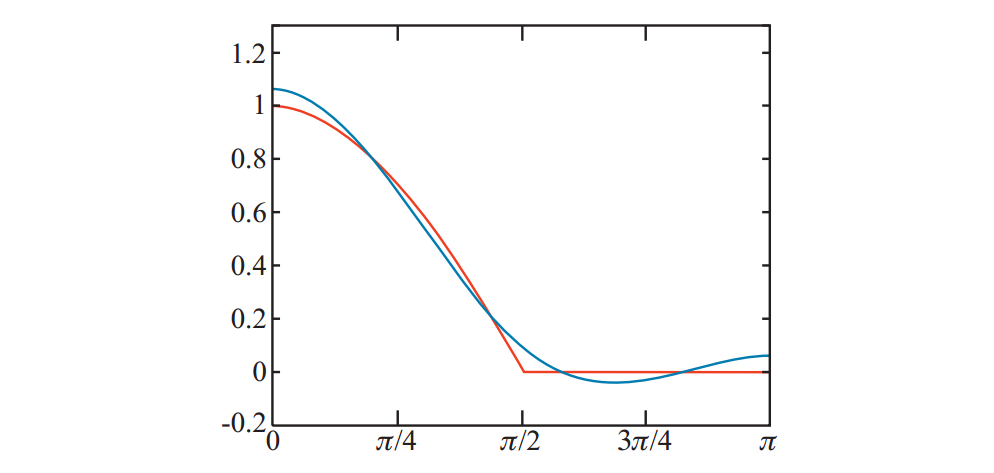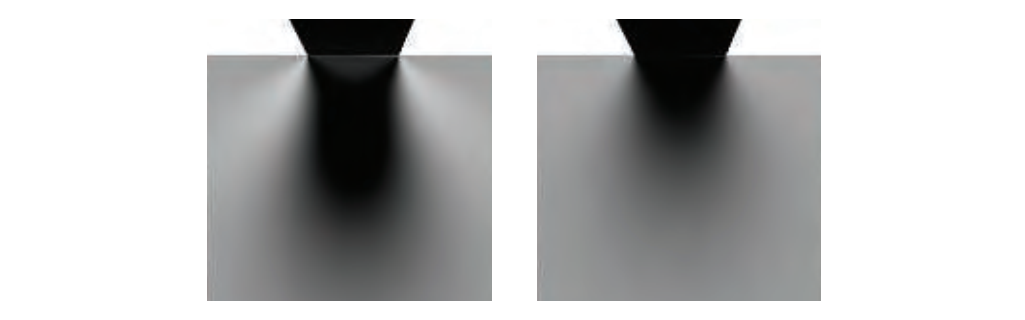$$k_{Lj}=\sum_tf_j(\boldsymbol{r}[t])L[t]d\omega[t],\tag{10.40}$$

$$k_{Ej}=k'_{\cos+j}k_{Lj}=k'_{\cos+j}\sum_tf_j(\boldsymbol{r}[t])L[t]d\omega[t],\tag{10.41}$$

#### 10.6.2 Other Representation（其他）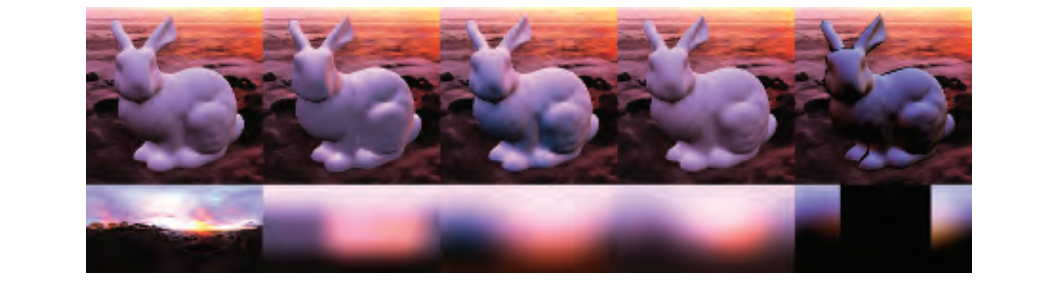$$E= \begin{cases} \pi((1-\frac12\sin\theta)L_{sky}+\frac12\sin\theta L_{ground}),;where;\theta\lt90^\circ,\\ \pi(\frac12\sin\theta L_{sky}+(1-\frac12\sin\theta) L_{ground}),;where;\theta\ge90^\circ, \end{cases}\tag{10.42}$$

$$E=\pi(\frac{1+\cos\theta}2L_{sky}+\frac{1-\cos\theta}2L_{ground}),\tag{10.43}$$

Forsyth提出了一种廉价而灵活的照明模型，称为trilight，它包括定向，双向，半球形和环绕照明作为特殊情况。

Valve最初引入了辐照度的环境立方体表示（第10.3.1节）。通常，我们在第10.3节中看到的所有球面函数表示都可用于预先计算的辐照度。对于辐照度函数所代表的低频信号，我们知道SH是一个很好的近似。我们倾向于创建特殊方法来简化或使用比球谐波更少的存储。

### 10.7 Sources of Error （误差来源）